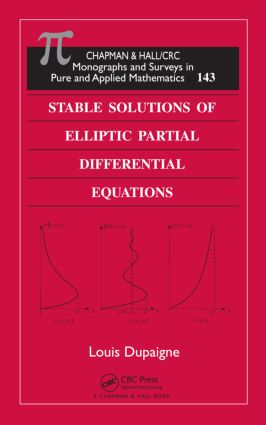# Stable Solutions of Elliptic Partial Differential Equations

## 1st Edition

Chapman and Hall/CRC

335 pages | 11 B/W Illus.

Paperback: 9780367382971
pub: 2019-07-31
SAVE ~\$14.99
Available for pre-order
\$74.95
\$59.96
x
Hardback: 9781420066548
pub: 2011-03-15
SAVE ~\$22.00
\$110.00
\$88.00
x
eBook (VitalSource) : 9780429150340
pub: 2011-03-15
from \$28.98

FREE Standard Shipping!

### Description

Stable solutions are ubiquitous in differential equations. They represent meaningful solutions from a physical point of view and appear in many applications, including mathematical physics (combustion, phase transition theory) and geometry (minimal surfaces).

Stable Solutions of Elliptic Partial Differential Equations offers a self-contained presentation of the notion of stability in elliptic partial differential equations (PDEs). The central questions of regularity and classification of stable solutions are treated at length. Specialists will find a summary of the most recent developments of the theory, such as nonlocal and higher-order equations. For beginners, the book walks you through the fine versions of the maximum principle, the standard regularity theory for linear elliptic equations, and the fundamental functional inequalities commonly used in this field. The text also includes two additional topics: the inverse-square potential and some background material on submanifolds of Euclidean space.

### Reviews

the author has put every effort into giving complete details of the computations. … the self-contained one hundred-page long appendices provide a splendid resource for the reader. The material contained there has been chosen with particular good taste and constitutes in itself a valuable source of information. Remarks and further features … are presented in an ‘exercise’ format. I have found many of them suitably posed and reasonable to work at the level of the book.

—José C. Sabina de Lis, Mathematical Reviews, 2012i

Defining Stability

Stability and the variations of energy

Linearized stability

Elementary properties of stable solutions

Dynamical stability

Stability outside a compact set

Resolving an ambiguity

The Gelfand Problem

Motivation

Dimension N = 1

Dimension N = 2

Dimension N ≥ 3

Summary

Extremal Solutions

Weak solutions

Stable weak solutions

The stable branch

Regularity Theory of Stable Solutions

Back to the Gelfand problem

Dimensions N = 1, 2,3

A geometric Poincaré formula

Dimension N = 4

Regularity of solutions of bounded Morse index

Singular Stable Solutions

The Gelfand problem in the perturbed ball

Flat domains

Partial regularity of stable solutions in higher dimensions

Liouville Theorems for Stable Solutions

Classifying stable entire solutions

Classifying solutions that are stable outside a compact set

A Conjecture of E De Giorgi

Statement of the conjecture

Motivation for the conjecture

Dimension N = 2

Dimension N = 3

Stability versus geometry of the domain

Symmetry of stable solutions

Beyond the stable branch

The parabolic equation

Other energy functional

Appendix A: Maximum Principles

Appendix B: Regularity Theory for Elliptic Operators

Appendix C: Geometric Tools

References

Index

Amiens, , France